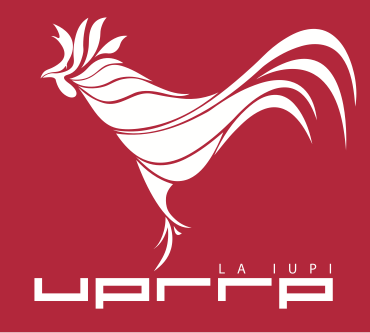## Seminar

COMBINATORIAL OPTIMIZATION: Packing Induced Stars in a Graph- An Extension of matching Theory,   Alexander Kelmans,   University of Puerto Rico,  Mon, 9 November, 2009,   2:30 p.m. A-207

### Abstract

We will consider the following optimization problem.

Given a graph G, ï¬nd a packing of induced stars of restricted size in G having the maximum number of vertices.

This problem is a natural generalization of the classical problem of ï¬nding in a graph a maximum matching.

We will show that all main results of matching theory can be extended to this problem. Namely, we will give a polynomial-time algorithm for solving this problem, the duality theorem, and the graph structure theorem (similar to that of Tutte-Berge duality theorem and Edmonds-Gallai structure theorem for matchings).

We will also discuss the matroid theory aspects of the above star packing problem.